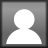# Fractions Part 6: Adding and Subtracting Fractions

Starting in 3rd grade, students start building understanding aboutadding and subtracting fractions by composing and decomposing simple fractions using concrete and visual models. Composing: 1/4 and 3/4 combine to make 4/4 (whole). Decomposing: 8/8 is made up of 2/8 and 6/8. In fourth grade, students begin to add and subtract fractions with like denominators, but should still be utilizing models, drawings, and number lines to illustrate and simplify. In fifth grade, students are expected to add and subtract fractions of all types (proper, improper, with unlike denominators, etc.).

With a firm foundation of composing and decomposing, partitioning, comparing, naming equivalent fractions, and understanding the relationship between certain fractions (such as halves / fourths / eighths / sixteenths; and thirds / ninths / sixths / twelfths; or fifths / tenths), then students are more prepared to perform operations with fractions. Here’s a great resource by Donna Boucher at Math Coach’s Corner: Composing and Decomposing Fractions activity on TPT (\$6)

Estimating: This is an important part of operations with fractions. Do you expect your answer to be less than 1/2, more than 1/2, more than 1? How do you know? If I was adding 8/9 + 11/12, my answer should be about _____? It should be slightly less than 2 because both of these fractions are almost 1.

If I am adding 4/6 and 6/8, my answer should be more than 1 because each of these fractions are greater than 1/2.

Different strategies: There are many “tricks” or shortcuts available to show students how to quickly add, subtract, or multiply fractions. I believe these shortcuts are only useful after a students has a strong understanding of why and how to find a common denominator and equivalent fraction. These shortcuts do not help build conceptual understanding of fractions.  I will focus on ways to understand the why using visual and pictorial models. Get your FREE copy of the following guides by clicking HERE.

Adding or subtracting fractions with like denominators: To make this clear to students that 1/4 + 1/4 does NOT equal 2/8, show with objects and pictures. Partition a whole to model this. Remember the best learning progression:  concrete and pictorial before abstract.Adding fractions with unlike denominators: Review my previous post Fractions Part 2 about drawing fractional parts, especially using bars or rectangles. This is a basic skill to making equivalent fractions (which is needed to add fractions with unlike denominators). While it might be tedious to draw pictures for each addition or subtraction problem, I recommend it as a way to introduce the concept so students see again that multiplying a fraction times a representative of 1 (2/2, 3/3, etc.) to name an equivalent fraction (to make it easier to add or subtract) does not change the shaded part, but it just divides the shaded part into smaller portions. Remember when dividing a whole number by 1 it does not change the answer – same concept for fractions.

Simplifying Fractions: I mentioned this some in part 5, but here it is again. The more students have practiced partitioning shapes they should start to internalize the relationship between some common fractions (to draw eighths, you might start with halves or fourths; to draw sixths or twelfths, you might start with thirds, etc.). Use the reverse process of removing lines from partitioned shapes to simplify.Using Prime Factorization to find the LCD/LCM and GCF: There’s a really good video on Learn Zillion to illustrate these steps below. Click here: Learn Zillion video

## 4 thoughts on “Fractions Part 6: Adding and Subtracting Fractions”

1.Christie Darling

It is interesting to read these posts for upper grades. I have taught 5th grade in the past and I see concepts that I taught but I now see a whole new way I could have taught them. Thanks for all of your hard work and dedication to the education of today’s students!

2.Cindy

Thank you! That is my goal – to provide strategies which make math more interesting, relevant, and understandable.

3.Rick Parks

Adding and Subtracting fractions maybe difficult at first but keeping on practicing will make it easier in the long run. Let me share you my proven method of learning fraction effectively.

To add and subtract fractions successfully is to make the rules stick to your memory.

Rules are:
Same denominator:
Add both numerators then reduce. The result would be the final answer.
Different denominator (4 steps):
1. Multiply the numerator of first fraction to the denominator of second fraction. The result is the new numerator of first fraction.
2. Multiply the numerator of the second fraction to the denominator of first fraction. The result is the new numerator of second fraction.
3. Multiply both denominators. The result is the common denominator for two fractions.

To make it stick to your memory:
Same numerator:
•Cindy Elkins